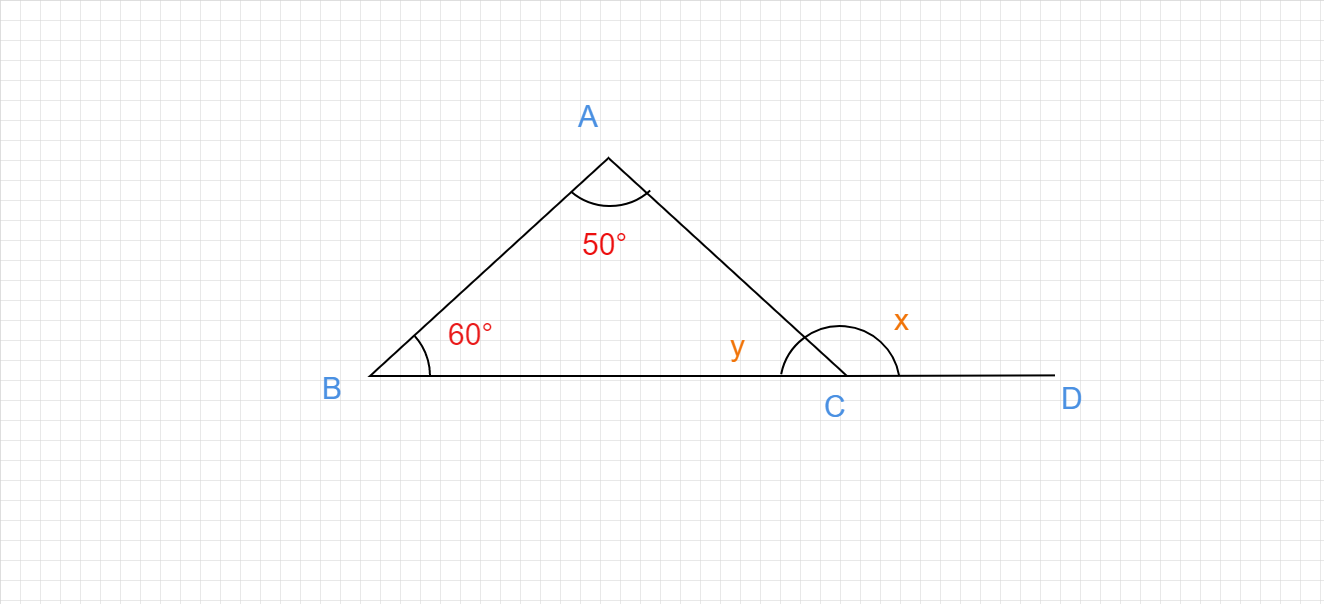"
">

# Find the values of x and y."

Given :

In the given triangle, two of the internal angles are 50° and 60°.

To do :

We have to find the values of x and y.

Solution :

We know that,

The sum of the angles in a triangle is 180°.

Therefore,

$50°+60°+y=180°$

$110°+y=180°$

$y=180°-110°$

$y=70°$

Angles x and y form a linear pair.

Therefore,

$x+y=180°$

$x+70°=180°$

$x=180°-70°$

$x=110°$.

The measure of angle x is 110° and y is 70°.

Updated on: 10-Oct-2022

33 Views